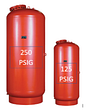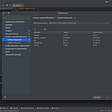# Importing NumPy

`import numpy as np`
`>>> import numpy as np>>> arr=np.array([1,2,3,4,5,6,7,7,8,9])>>> print(arr)[1 2 3 4 5 6 7 7 8 9]>>> arr>>> array([1, 2, 3, 4, 5, 6, 7, 7, 8, 9])>>> arr.dtypedtype('int32')>>> print("No. of dimensions: ", arr.ndim)No. of dimensions:  1>>> print("Shape of array: ", arr.shape)Shape of array:  (10,)>>> print("Size of array: ", arr.size)Size of array:  10>>> a=np.array(1,2,3,4,5) #INCORRECT WAY Traceback (most recent call last):  File "<stdin>", line 1, in <module>ValueError: only 2 non-keyword arguments accepted`
`>>>import Numpy as np>>>array = np.arange(10)# ONE DIMENSIONAL ARRAY>>>array>>>array([0,  1,  2,  3,  4, 5,  6,  7,  8,  9])#MULTI-DIMENSIONAL ARRAY>>>array = np.arange(10).reshape(2,5)>>>arrayarray([[ 0,  1,  2,  3,  4],       [ 5,  6,  7,  8,  9]])#It will be craete 10 intergers and then convert the array into a two-dimensional array with 2rows and 5 columns.`
`>>>np.zeros((4,5))array([[0., 0., 0., 0., 0.],       [0., 0., 0., 0., 0.],       [0., 0., 0., 0., 0.],       [0., 0., 0., 0., 0.]])`
`>>>np.ones((4,5))array([[1,1,1,1,1],          [1,1,1,1,1],          [1,1,1,1,1],          [1,1,1,1,1]])`
`>>> np.empty((4,5))array([[6.23042070e-307, 4.67296746e-307, 1.69121096e-306,        3.11522054e-307, 1.42413555e-306],       [1.78019082e-306, 1.37959740e-306, 6.23057349e-307,        1.02360935e-306, 1.69120416e-306],       [1.78022342e-306, 6.23058028e-307, 1.06811422e-306,        1.33508761e-307, 1.78022342e-306],       [1.05700345e-307, 1.11261977e-306, 1.69113762e-306,        1.33511562e-306, 2.18565567e-312]])`
`>>> np.linspace(0,5,10)array([0.        , 0.55555556, 1.11111111, 1.66666667, 2.22222222,       2.77777778, 3.33333333, 3.88888889, 4.44444444, 5.        ])`
`SYNATX: numpy.ravel(array, order = 'C')`
`SYNTAX: numpy.flatten(array,order='C')`
`import numpy as npy = np.array(((1,2,3),(4,5,6),(7,8,9)))OUTPUT:print(y.flatten())[1   2   3   4   5   6   7   8   9]print(y.ravel())[1   2   3   4   5   6   7   8   9]`
• `flatten` always returns a copy.
• `ravel` returns a view of the original array whenever possible. This isn't visible in the printed output, but if you modify the array returned by ravel, it may modify the entries in the original array. If you modify the entries in an array returned from flattening this will never happen. ravel will often be faster since no memory is copied, but you have to be more careful about modifying the array it returns.

# Indexing and slicing arrays

`>>>import numpy as np>>>arr=np.array([0,1,2,3,4,5,6,7,8,9])>>>arr # output: 8>>>arr.reshape(2,5)>>>arrarray([[0,1,2,3,4],       [5,6,7,8,9]])Note : Our array is temporarily changed.>>>arr=arr.reshape(2,5)#this changes our one-dimensional array to two-dimensional array permanently.>>>arr[1,4]# output: 9`
• the first index selects the row
• the second index selects the column
• The first index, i, selects the matrix
• The second index, j, selects the row
• The third index, k, select the column
`>>> arr[1:8]array([1, 2, 3, 4, 5, 6, 7])>>>arr=arr.reshape(2,5)>>> arr[1:,2:4]array([[7, 8]])`

# Linear Algebra

• rank, determinant, trace, etc. of an array.
• eigenvalues of matrices
• matrix and vector products (dot, inner, outer, etc. product), matrix exponentiation
• solve linear or tensor equations and much more!
`>>> A = np.array([[ 1, 2 ,3], [ 4, 5 ,6]])>>> B = np.array([7,8,9]) >>> Aarray([[1, 2, 3],       [4, 5, 6]])>>> Barray([7, 8, 9])>>> A.shape(2, 3)>>> B.shape(3,)>>> A.Tarray([[1, 4],       [2, 5],       [3, 6]])>>> >>> B.Tarray([7, 8, 9])>>> >>> A.dot(B)array([ 50, 122])>>> >>> np.dot(A,B)`

# Ax = b : numpy.linalg

`>>> import numpy as np>>> from numpy.linalg import solve>>> A = np.array([[1,2],[3,4]])>>> Aarray([[1, 2],       [3, 4]])>>> b = np.array([10, 20])>>> b>>> x = solve(A,b)>>> xarray([ 0.,  5.])>>> a = np.array([[3,-9],[2,5]])>>> np.linalg.det(a)33.000000000000014`
`>>>from numpy.linalg import eig>>> arr=np.array([[1,2],[3,4]])>>> arrarray([[1, 2],       [3, 4]])>>> eig(arr)(array([-0.37228132,  5.37228132]), array([[-0.82456484, -0.41597356],       [ 0.56576746, -0.90937671]]))`

--

--

--

## More from Sweta Barnwal

3rd year CSE student at IIITKALYANI , enthusiastic learner and explorer

Love podcasts or audiobooks? Learn on the go with our new app.

## Vector Icons for Xamarin.Form, add 7000+ icons to your project with a single package installation.## Hydronic Expansion Tank Location in High Rise Projects (Part 3)## How to Handle Bad Data in Spark SQL## Review of an ARM-based cloud server## How to run GUI Software in Docker## One codebase, One helm repository## C++ Development Tutorial 4: Static and Dynamic Libraries## Sweta Barnwal

3rd year CSE student at IIITKALYANI , enthusiastic learner and explorer

## Implement C++ function template, Function Overloading & do-while loop in Python## Different ways of creating Numpy Arrays in Python## OLD Man Monitoring System using Open CV & MediaPipe in Python## [OpenCV] Basics review notes (1) & PyCharm unboxing